## Overview: Decomposition Algorithm

The SAS/OR decomposition algorithm (DECOMP) provides an alternative method for solving linear programs (LPs) and mixed integer linear programs (MILPs) by exploiting the ability to efficiently solve a relaxation of the original problem. The algorithm is available as an option in the OPTMODEL, OPTLP and OPTMILP procedures and is based on the methodology described in Galati (2009).

A standard linear or mixed integer linear program has the formulation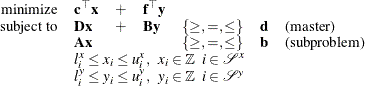where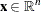is the vector of structural variables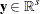is the vector of master-only structural variables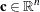is the vector of objective function coefficients that are associated with variables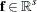is the vector of objective function coefficients that are associated with variables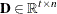is the matrix of master constraint coefficients that are associated with variables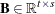is the matrix of master constraint coefficients that are associated with variables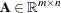is the matrix of subproblem constraint coefficients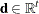is the vector of master constraints’ right-hand sides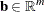is the vector of subproblem constraints’ right-hand sides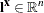is the vector of lower bounds on variables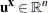is the vector of upper bounds on variables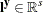is the vector of lower bounds on variables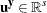is the vector of upper bounds on variables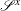is a subset of the set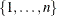of indices on variables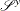is a subset of the set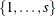of indices on variablesA relaxation of the preceding mathematical program can be formed by removing the master constraints, which are defined by the matrices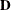and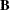. The resulting constraint system, defined by the matrix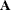, forms the subproblem, which can often be solved much more efficiently than the entire original problem. This is the one of the key motivators for using the decomposition algorithm.

The decomposition algorithm works by finding convex combinations of extreme points of the subproblem polyhedron that satisfy the constraints defined in the master. For MILP subproblems, the strength of the relaxation is another important motivator for using this method. If the subproblem polyhedron defines feasible solutions that are close to the original feasible space, the chance of success for the algorithm increases.

The region that defines the subproblem space is often separable. That is, the formulation of the preceding mathematical program can be written in block-angular form as follows: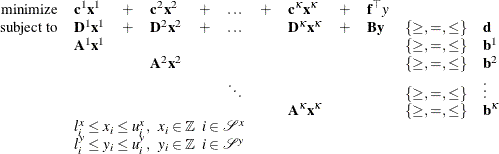where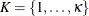defines a partition of the constraints (and variables) into independent subproblems (blocks) such that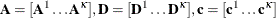, and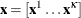. This type of structure is fairly common in modeling mathematical programs. For example, consider a model that defines a workplace with separate departmental restrictions (defined as the subproblem constraints), which are coupled together by a company-wide budget across departments (defined as the master constraint). By relaxing the budget (master) constraint, the decomposition algorithm can take advantage of the fact that the decoupled subproblems are separable, and it can process them in parallel. A special case of block-angular form, called block-diagonal, occurs when the set of master constraints is empty. In this special case, the subproblem matrices define the entire original problem.

An important indicator of a problem that is well suited for decomposition is the amount by which the subproblems cover the original problem with respect to both variables and constraints in the original presolved model. This value, expressed as a percentage of the original model is known as the coverage. For LPs, the decomposition algorithm usually performs better than standard approaches only if the subproblems cover a significant amount of the original problem. For MILPs, the correlation between performance and coverage is more difficult to determine, because the strength of the subproblem with respect to integrality is not always proportional to the size of the system. Regardless, it is unlikely that the decomposition algorithm outperforms more standard methods (such as branch-and-cut) for problems with small coverage.

The primary input and output for the decomposition algorithm are identical to those needed and produced by the OPTLP, OPTMILP, and OPTMODEL procedures. For more information, see the sections Data Input and Output, Data Input and Output, Details: LP Solver, and Details: MILP Solver. The only additional input that can be provided for the decomposition algorithm is an explicit definition of the partition of the subproblem constraints. The following section gives a simple example of providing this input for both PROC OPTMILP and PROC OPTMODEL.